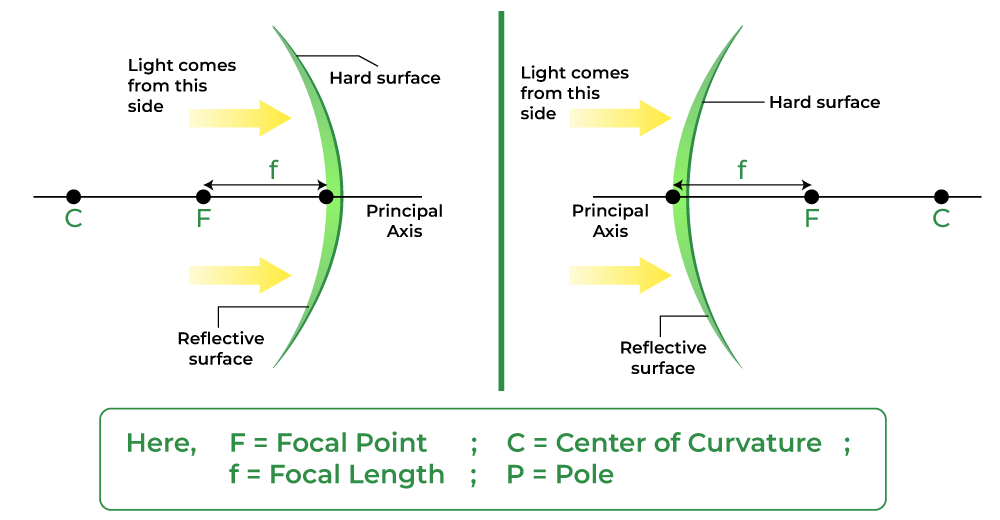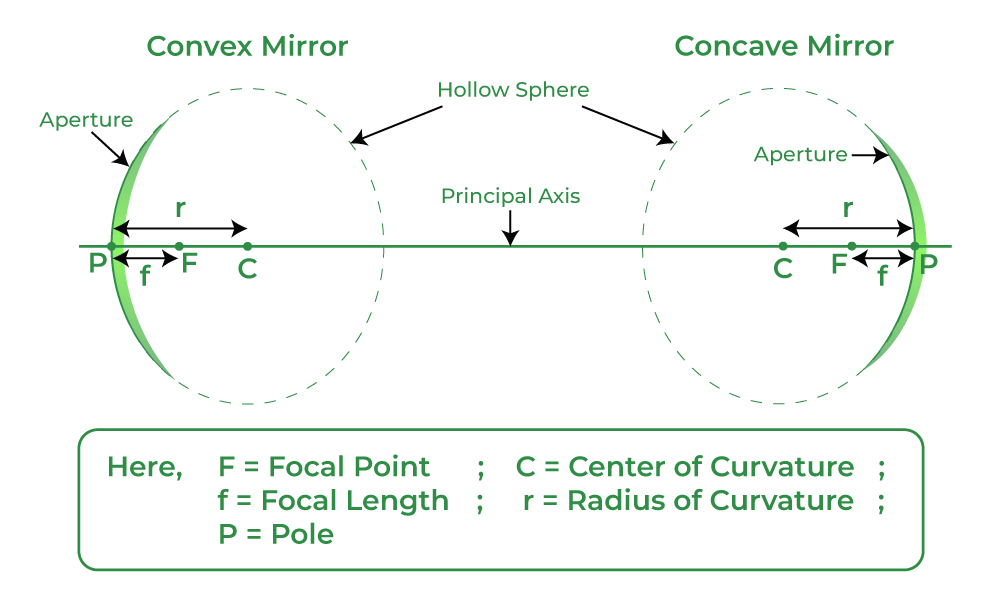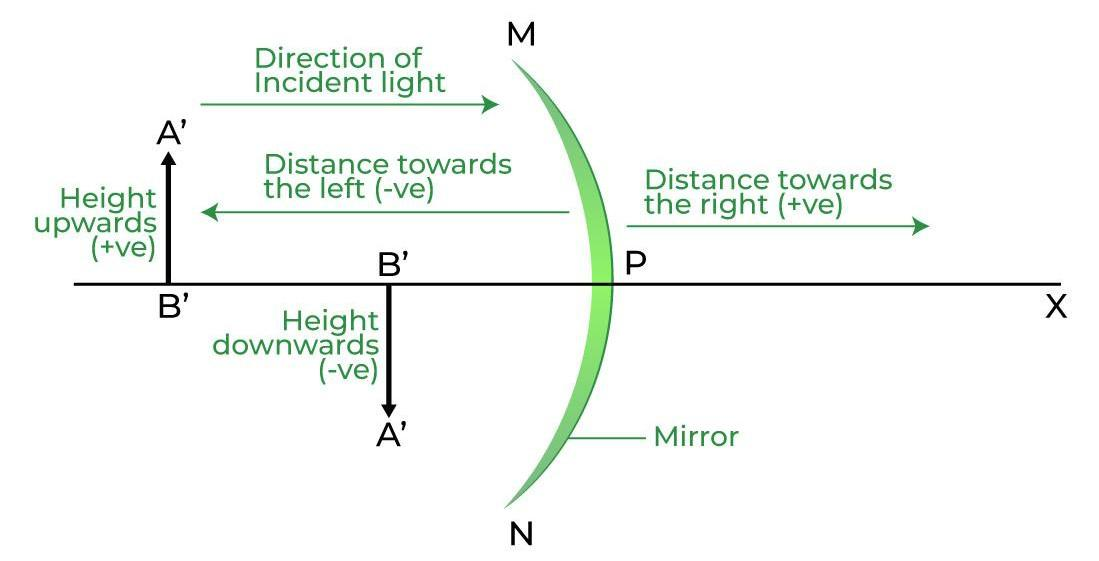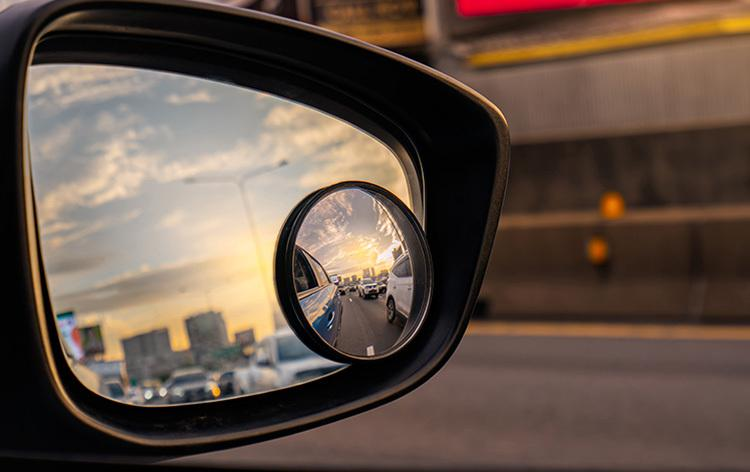GFG App
Open AppBrowser
Continue

Spherical mirrors are generally constructed from glass. A spherical surface is a part cut from a hollow sphere. This curved surface of the glass has a silver coating on one side and a polished surface on the other, where the reflection of light takes place. The term “convex mirror” refers to a mirror where the reflection occurs at the convex surface, and the term “concave mirror” refers to a mirror where the reflection occurs at the concave surface. The passenger-side wing mirrors of automobiles are the most common examples of convex mirrors. Despite having wider fields of view than equivalent flat mirrors, these kinds of mirrors tend to make objects appear farther away and smaller than they actually are.

## Spherical Mirrors

A spherical mirror or a mirror that is a part of a sphere is a mirror that has the shape of a piece that is cut out of a spherical surface or material. There exist two types of spherical mirrors which are: Concave and Convex mirrors

## Spherical Mirror Formula

The spherical mirror formula is a relation that describes how object distance (u) and image distance (v) are related to the focal length (f) of a spherical mirror. The spherical mirror equation is one of the most important relations from optics in Physics.

The spherical mirror formula is given as,Here,

f is the focal length of the mirror, which is the distance from the Principal Focus and the Pole of the spherical mirror.

v is the image distance of the mirror, which is the distance from the image formed and the pole of the spherical mirror.

u is the object distance of the mirror, which is the distance between the object and the pole of the spherical mirror.

The focal length of the mirror is equal to half of the radius of curvature of the spherical mirror and is given by the relation:where,

f is the focal length of the spherical mirror and

R is the Radius of Curvature of the spherical mirror.

Magnification of the spherical mirror, determines how smaller or bigger the image is formed after reflection from the spherical mirror. Magnification is given either by the ratio of image and object height or by the ratio of image and object distance of the mirror.where,

I is the Height of the Image formed,

O is the Height of the Object,

v is the image distance and

u is the object distance.

## History of Spherical Mirrors in Human Civilization

As early as 30,000 years ago, people used spherical mirrors to collect water in prehistoric containers or reflect items in quiet, dark water (or utensils). The first mirrors were made from polished volcanic glass, such as obsidian, and are the oldest examples of produced mirrors. Mirrors made of obsidian that date to roughly 6000 BC have been discovered in Anatolia (now Turkey). Around 3000 BC and 4000 BC, respectively, polished copper mirrors were created in Mesopotamia and ancient Egypt.

Spherical mirrors have been utilized by human civilization for a very long time, not just in those areas. Polished stone mirrors from the year 2000 BC have also been found in Central and South America. Since 2000 BC, China has been making bronze mirrors.

## Basic Terminologies for Spherical Mirrors

There are some common terms that we need to know while studying spherical mirrors, and they are as follows:• Centre of Curvature: The point in the centre of the mirror surface that passes through the curve of the mirror and has the same tangent and curvature at that point. It is represented by the capital letter C.
• Radius of Curvature: It is considered the linear distance between the pole and the centre of curvature. It is represented by the capital letter R, R=2f
• Principal axis: An imaginary line that passes through the optical centre and from the centre of curvature of a spherical mirror. All the measurements are based on this line.
• Pole: The midpoint or the centre point of the spherical mirror. It is represented by capital P. All the measurements are made from it only.
• Aperture: An aperture of a mirror is a point from which the reflection of light actually takes place or happens. It also gives an idea about the size of the mirror.
• Principal Focus: Principal Focus can be called the Focal Point also. It is present on the axis of a mirror where the rays of light parallel to the principal axis converge or appear to converge or diverge after reflection.
• Focus: It is any given point on the principal axis where light rays parallel to the principal axis will converge or appear to converge after getting reflected from the mirror.

## Structure of Spherical Mirrors

A spherical mirror can be a concave or a convex mirror depending upon the surface of the reflection. If it is bulged out then it is a convex spherical mirror whereas if it is bent inwards it is termed as a concave spherical mirror.A typical spherical mirror is a part of a big sphere of which the cut-out has been taken. The following diagram describes different parts which are there in a spherical mirror. The definition of these parts is already given above.

## Types of Spherical Mirrors

Spherical Mirrors are of two types, namely:

1. Concave Mirrors
2. Convex Mirrors

The two types of spherical mirrors are discussed in detail:

### Concave Mirror

A spherical mirror of which the reflecting surface is curved inwards which means that it faces towards the centre of the sphere is known to be a concave mirror. The back of the mirrors is always shaded so that reflection can take place only from the inward bulged surface.

The surface of the spoon which is curved inwards can be approximated to a concave mirror. It is also known as the converging mirror as the ray of light after bouncing back from it appears to converge at some points where we can obtain a real, inverted, and enlarged or diminished image based on the location of the object.

Uses of Concave Mirror

• Converging mirrors are most widely used in shaving because they have reflective and curved surfaces.
• A concave mirror is used in the ophthalmoscope
• These mirrors are also widely used in making astronomical telescopes. In an astronomical telescope, a converging mirror of a diameter of about 5 meters or more is used as the objective.
• Converging mirrors are widely used in headlights of automobiles and in motor vehicles, torchlights, railway engines, etc. as reflectors.
• Large converging mirrors are used to focus the sunlight to produce heat in the solar furnace.

### Convex Mirror

A spherical mirror having its reflecting surface curved outwards is known to be a convex mirror. The back of the mirror is shaded so that reflection only takes place from the outward bulged part. The surface of the spoon which is bulged outwards can be assumed to be a convex mirror. It is also known as a diverging mirror as the light after reflecting through its surface diverges to many directions but appears to meet at some points where the virtual, erect image of diminished size is formed.

Uses of Convex Mirror

• Convex mirrors are used inside buildings so that people can see all around the building at once.
• The convex mirror is used in vehicles. Convex mirrors are commonly used as rear-view mirrors in the case of automobiles and vehicles because they can diverge light beams and make virtual images.
• These mirrors are mostly used for constructing magnifying glasses. In industries, to construct a magnifying glass, two convex mirrors are placed back to back.
• Diverging mirrors are also used for security purposes in many places. They are placed near ATMs to let the bank customers check whether someone is behind them or not.
• Convex mirrors are also widely used in various other places for example streetlight reflectors because they can spread light over bigger areas.

## Sign Conventions for Spherical Mirrors

A set of rules that are used to set signs for terms like the object distance, image distance, focal length, etc used in spherical mirrors for mathematical analysis during the image formation are called the Sign Conventions for Spherical Mirrors.According to the sign convention for spherical mirrors:

1. All distances are measured or taken from the pole of the spherical mirror.
2. Objects are considered to be placed on the left side of the spherical mirror.
3. The distances measured along the direction of the incident ray are taken as positive while, the distance measured along the direction of the reflected ray or opposite is taken as negative.

## Image Formation by Spherical Mirrors

Image formed by any type of mirror can be found either where the reflected light appears to diverge from or where it converges. We have two types of spherical mirrors Concave and Convex Mirrors. Let’s discuss the image formation in each type of mirror as:

Properties of the Image formed by Concave Mirrors

• Point-sized image, highly diminished in size, Real and inverted image.
• The parallel lines which come from the very distant object at infinity after striking the reflecting surface of the concave mirror get reflected back and meet at a point, or we can say in this case converge at a point. This point is known as the principal focus of the concave mirror.

Properties of the Image formed by Convex Mirrors

• The image formed is highly diminished in size, virtual, and erect.
• The parallel lines which come from the very distant object at infinity after striking the reflecting surface of the convex mirror get reflected back and appear to meet at a point, or we can say in this case diverge from the surface and appear to meet at a point. This point is known as the principal focus of a convex mirror.

## Uses of Spherical Mirrors

• Concave mirrors are used torch headlights to disperse light over a larger surface, hence enhancing the field of vision.
• Convex mirrors are used in car’s rearview mirror, as it gives a wider field of view, that helps the driver to see most of the traffic behind him.• Concave mirrors are used in solar cookers as reflectors to focus sunlight on a specific area and raise the box’s temperature.
• Convex mirrors are used in the rear-view mirror to provide a larger view of the road and oncoming traffic.
• Concave mirrors are used in telescopes, satellite dishes, and by dentists and ENT specialists to create images of the teeth, ears, skin, and other body parts that are larger than the actual.## FAQs on Spherical Mirrors

Question 1: Write Daily Life Applications of Spherical Mirrors.

Daily Life Applications of Spherical Mirrors are,

• In Rear-view Mirrors of automobiles to see behind the car, etc.
• In security mirrors used in banks, ATMs, stores, etc.
• Satellites uses concave mirrors to receive and enhance signals.
• Concave mirrors are used in solar cookers as reflectors to focus sunlight on a specific area and raise the temperature of the box.

Question 2: How many types of spherical mirrors are there? Name them.

There are two types of spherical mirrors, they are:

1. Concave Mirror
2. Convex Mirror

Question 3: Define the term “radius of curvature” for spherical mirrors.

Radius of Curvature: is considered the linear distance between the pole and the centre of curvature. It is represented by the capital letter R,

And it is related to focal length length f as,

R = 2f

Question 4: How can you define the principal focus of a concave mirror?

When a parallel beams of light rays are incident on a concave mirror they after reflection through its surface converge at a particular point on the principal axis which is known as principal focus of concave mirror.

Question 5: How can you define the principal focus of a convex mirror?

When a parallel beams of light rays are incident on a convex mirror they after reflection through its surface diverge through its surface but appear to meet at a particular point on the principal axis which is known as principal focus of convex mirror.

Question 6: What is Pole in Spherical Mirrors?